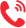学校：
地区：

北京大学
• 专业名称
• 所属学院
• 学制
• 学费(元)
• 地区
• 简章详情
• 报名
中国人民大学
• 专业名称
• 所属学院
• 学制
• 学费(元)
• 地区
• 简章详情
• 报名
清华大学
• 专业名称
• 所属学院
• 学制
• 学费(元)
• 地区
• 简章详情
• 报名
北京航空航天大学
• 专业名称
• 所属学院
• 学制
• 学费(元)
• 地区
• 简章详情
• 报名
北京理工大学
• 专业名称
• 所属学院
• 学制
• 学费(元)
• 地区
• 简章详情
• 报名
大连理工大学
• 专业名称
• 所属学院
• 学制
• 学费(元)
• 地区
• 简章详情
• 报名
东北大学
• 专业名称
• 所属学院
• 学制
• 学费(元)
• 地区
• 简章详情
• 报名
哈尔滨工业大学
• 专业名称
• 所属学院
• 学制
• 学费(元)
• 地区
• 简章详情
• 报名
复旦大学
• 专业名称
• 所属学院
• 学制
• 学费(元)
• 地区
• 简章详情
• 报名
同济大学
• 专业名称
• 所属学院
• 学制
• 学费(元)
• 地区
• 简章详情
• 报名
上海交通大学
南京大学
• 专业名称
• 所属学院
• 学制
• 学费(元)
• 地区
• 简章详情
• 报名
东南大学
• 专业名称
• 所属学院
• 学制
• 学费(元)
• 地区
• 简章详情
• 报名
浙江大学
• 专业名称
• 所属学院
• 学制
• 学费(元)
• 地区
• 简章详情
• 报名
厦门大学
• 专业名称
• 所属学院
• 学制
• 学费(元)
• 地区
• 简章详情
• 报名
山东大学
• 专业名称
• 所属学院
• 学制
• 学费(元)
• 地区
• 简章详情
• 报名
武汉大学
• 专业名称
• 所属学院
• 学制
• 学费(元)
• 地区
• 简章详情
• 报名
华中科技大学
• 专业名称
• 所属学院
• 学制
• 学费(元)
• 地区
• 简章详情
• 报名
中南大学
• 专业名称
• 所属学院
• 学制
• 学费(元)
• 地区
• 简章详情
• 报名
中山大学
• 专业名称
• 所属学院
• 学制
• 学费(元)
• 地区
• 简章详情
• 报名
华南理工大学
• 专业名称
• 所属学院
• 学制
• 学费(元)
• 地区
• 简章详情
• 报名
四川大学
• 专业名称
• 所属学院
• 学制
• 学费(元)
• 地区
• 简章详情
• 报名
电子科技大学
• 专业名称
• 所属学院
• 学制
• 学费(元)
• 地区
• 简章详情
• 报名
西安交通大学
• 专业名称
• 所属学院
• 学制
• 学费(元)
• 地区
• 简章详情
• 报名
西北工业大学
• 专业名称
• 所属学院
• 学制
• 学费(元)
• 地区
• 简章详情
• 报名
兰州大学
• 专业名称
• 所属学院
• 学制
• 学费(元)
• 地区
• 简章详情
• 报名
北京科技大学
• 专业名称
• 所属学院
• 学制
• 学费(元)
• 地区
• 简章详情
• 报名
北京邮电大学
• 专业名称
• 所属学院
• 学制
• 学费(元)
• 地区
• 简章详情
• 报名
河北工业大学
• 专业名称
• 所属学院
• 学制
• 学费(元)
• 地区
• 简章详情
• 报名
华东理工大学
• 专业名称
• 所属学院
• 学制
• 学费(元)
• 地区
• 简章详情
• 报名
东华大学
• 专业名称
• 所属学院
• 学制
• 学费(元)
• 地区
• 简章详情
• 报名
南京理工大学
• 专业名称
• 所属学院
• 学制
• 学费(元)
• 地区
• 简章详情
• 报名
暨南大学
• 专业名称
• 所属学院
• 学制
• 学费(元)
• 地区
• 简章详情
• 报名
广西大学
• 专业名称
• 所属学院
• 学制
• 学费(元)
• 地区
• 简章详情
• 报名
贵州大学
• 专业名称
• 所属学院
• 学制
• 学费(元)
• 地区
• 简章详情
• 报名
云南大学
• 专业名称
• 所属学院
• 学制
• 学费(元)
• 地区
• 简章详情
• 报名
西北大学
• 专业名称
• 所属学院
• 学制
• 学费(元)
• 地区
• 简章详情
• 报名
天津财经大学
• 专业名称
• 所属学院
• 学制
• 学费(元)
• 地区
• 简章详情
• 报名
江西理工大学
• 专业名称
• 所属学院
• 学制
• 学费(元)
• 地区
• 简章详情
• 报名
云南财经大学
• 专业名称
• 所属学院
• 学制
• 学费(元)
• 地区
• 简章详情
• 报名
西安理工大学
• 专业名称
• 所属学院
• 学制
• 学费(元)
• 地区
• 简章详情
• 报名
长江商学院
• 专业名称
• 所属学院
• 学制
• 学费(元)
• 地区
• 简章详情
• 报名
陕西工商管理硕士学院
• 专业名称
• 所属学院
• 学制
• 学费(元)
• 地区
• 简章详情
• 报名

上海交通大学
• 专业名称
• 所属学院
• 学制
• 学费(元)
• 地区
• 简章详情
• 报名

更多 >>

更多 >>

## 联系方式400-101-7010

报名咨询热线

400-101-7010